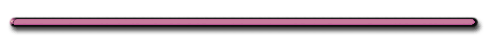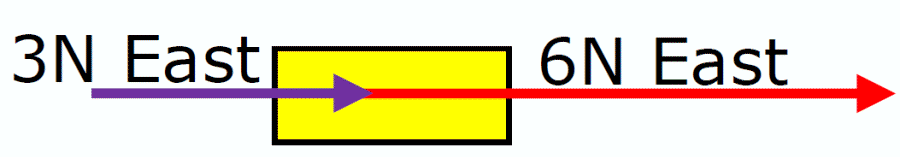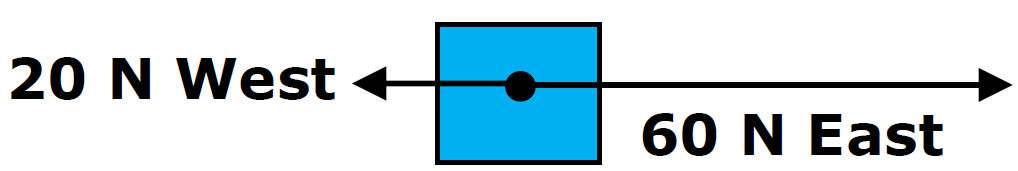HomeWhen doesn't 100 + 100 = 200?

Video Instruction

showmethephysics.com

B. Combining Force Vectors

Result
ant- single vector that will have the same effect as two or more vectors

 2 + 2 = 4 Right???   Sometimes !!!!! Not always true  with vectorsSometimes 2 + 2 = 0

1) Finding Resultants.

Case I - Forces acting in same direction

(Angle = 0 degrees)Resultant = 9 N East

Is resultant different than the one above?RULE when Ө =  0° Add Forces

Case II - Forces acting in opposite direction (Angle = 180°)Resultant = 40 N East

 If  Ө  = 180° Subtract smaller vector from largerRange of Resultants